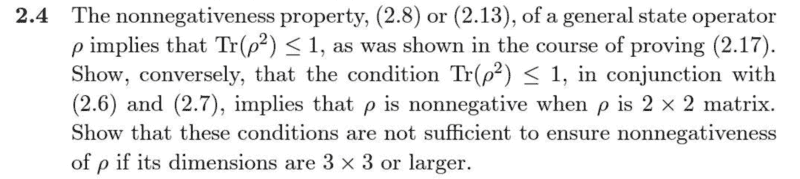# Question from Ballentine regarding nonnegativeness property of a general state operator

EE18
I am trying to solve Problem 2.4 in Ballentine:I note in my attempt below to what (2.6) and (2.7) refer.

My attempt thus far is as follows:
A ##2 \times 2## state operator can be represented in a particular orthonormal ##\beta = \{\phi_i\}## as below, where we have enforced trace normalization (2.6) and self-adjointness (2.7) (and have yet to enforce nonnegativeness),
$$[\rho]_{\beta} = \begin{bmatrix} a & b \\ b^* & (1-a) \end{bmatrix}$$
Now enforcing ##Tr{\rho^2}## and using the basis independence of the trace we obtain
$$Tr{\rho^2} = Tr{[\rho]_{\beta}^2} = Tr{ \begin{bmatrix} a & b \\ b^* & (1-a) \end{bmatrix}^2} = a^2 +2|b|^2+ (1-a)^2 \leq 1$$
with ##a \in \mathbb{R}##.

Now for an arbitrary ##u## in our space we may expand ##u = \sum_i c_i {\phi_i}## so we can immediately compute
$$(u,\rho u) = \begin{bmatrix} c_1^* & c_2^* \end{bmatrix}\begin{bmatrix} a & b \\ b^* & (1-a) \end{bmatrix}\begin{bmatrix} c_1 \\ c_2 \end{bmatrix} = \begin{bmatrix} c_1^* & c_2^* \end{bmatrix} \begin{bmatrix} ac_1 + bc_2 \\ b^*c_1+(1-a)c_2 \end{bmatrix}$$
$$=c_1^*(ac_1 + bc_2)+ c_2^*(b^*c_1+(1-a)c_2) = |{c_1}|^2a +2\textrm{Re}(c_1^*c_2b) + (1-a)^2|{c_2}|^2$$
but I can't seem to see how to go further here. It seems like I have to use my aforementioned inequality but I can't see how. Any help would be greatly appreciated.

For this type of problem, it's often more efficient to work with the eigenvalues directly, rather than a generic matrix. For this problem, we are given that:
$$Tr(\rho^2) ~\le 1 ~,~~~~ Tr(\rho) ~=~ 1 ~,~~~~ \rho = \rho^\dagger ~.$$For the 2D case, there are 2 eigenvalues, ##\rho_1## and ##\rho_2##, say, hence the eigenvalues of ##\rho^2## are the squares of these.

Self-adjointness of ##\rho## implies both the ##\rho_i## are real, hence ##\,\rho_i^2 \ge 0##.

The trace of a matrix is the sum of its eigenvalues, so we have 2 conditions:
$$\rho_1 + \rho_2 ~=~ 1 ~,~~~~ \rho^2_1 + \rho^2_2 ~\le~ 1 ~.$$Squaring the 1st equation gives $$\rho_1^2 + \rho_2^2 + 2 \rho_1 \rho_2 ~=~ 1 ~,$$and using this in conjunction with the 2nd equation implies... what?

I leave it to you to figure out the rest of the proof, including the follow-on of why it doesn't work for higher dimensional matrices.•DrClaude, vanhees71 and EE18
EE18
For this type of problem, it's often more efficient to work with the eigenvalues directly, rather than a generic matrix. For this problem, we are given that:
$$Tr(\rho^2) ~\le 1 ~,~~~~ Tr(\rho) ~=~ 1 ~,~~~~ \rho = \rho^\dagger ~.$$For the 2D case, there are 2 eigenvalues, ##\rho_1## and ##\rho_2##, say, hence the eigenvalues of ##\rho^2## are the squares of these.

Self-adjointness of ##\rho## implies both the ##\rho_i## are real, hence ##\,\rho_i^2 \ge 0##.

The trace of a matrix is the sum of its eigenvalues, so we have 2 conditions:
$$\rho_1 + \rho_2 ~=~ 1 ~,~~~~ \rho^2_1 + \rho^2_2 ~\le~ 1 ~.$$Squaring the 1st equation gives $$\rho_1^2 + \rho_2^2 + 2 \rho_1 \rho_2 ~=~ 1 ~,$$and using this in conjunction with the 2nd equation implies... what?

I leave it to you to figure out the rest of the proof, including the follow-on of why it doesn't work for higher dimensional matrices.Thank you so much for the detailed response. If possible, I came up with a demonstration of why it doesn't work for ##\dim V > 2## but it's really ugly:

In the case of 3 or more dimensions (for arbitrary dimension consider a state operator with 3 nonzero eigenvalues) we see that we can follow the proof up to the point ##Tr{\rho^2} = \rho_1^2+ \rho_2^2 + \rho_3^2 \leq 1 = \rho_1^2+ \rho_2^2 \rho_3^2 +2\rho_1 \rho_2 +2\rho_1 \rho_3 +2\rho_3 \rho_2## which implies ##\rho_1 \rho_2 +\rho_1 \rho_3 +\rho_3 \rho_2 \geq 0##. We can imagine obeying this constraint with one negative eigenvalue and two positive eigenvalues such that the positive eigenvalues "outweigh" the negative. Consider ##\rho_1 = \rho_2 -1/10 = 1/2## and ##\rho_3 = -1/10##. Then we have ##\rho_1 \rho_2 +\rho_1 \rho_3 +\rho_3 \rho_2 \geq 0## and ##Tr{\rho} = 1##. If we then take the eigenvector corresponding to that negative eigenvalue we see that the expectation value is negative.

Would you be able to suggest a nicer proof? Thank you again!

•